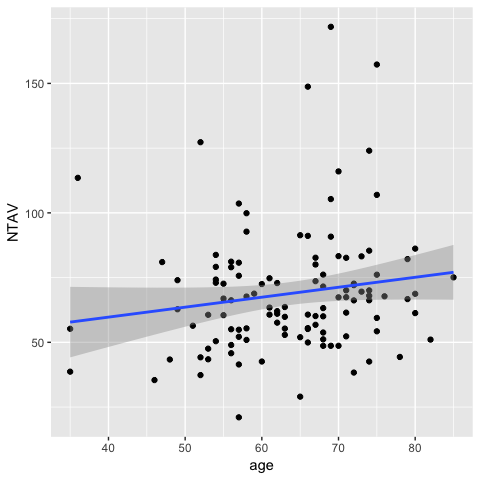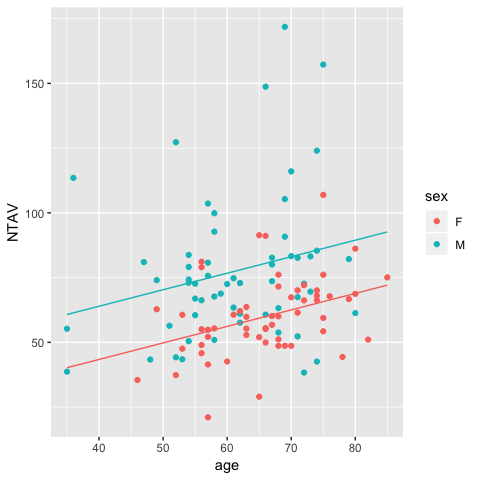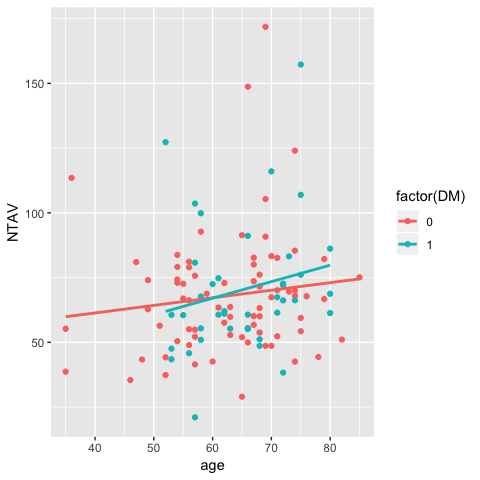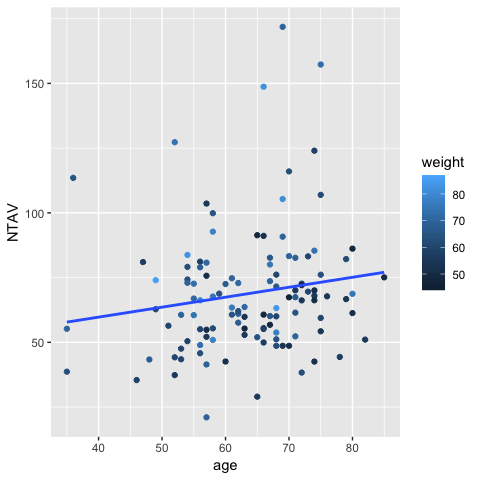# ggPredict() - Visualize multiple regression model

#### 2018-07-22

``````To reproduce this document, you have to install R package ggiraphExtra from github.
install.packages("devtools")
devtools::install_github("cardiomoon/ggiraphExtra")``````

# Linear regression Model

## Simple linear regression model

In univariate regression model, you can use scatter plot to visualize model. For example, you can make simple linear regression model with data `radial` included in package moonBook. The radial data contains demographic data and laboratory data of 115 pateints performing IVUS(intravascular ultrasound) examination of a radial artery after tansradial coronary angiography. The NTAV(normalized total atheroma volume measured by intravascular ultrasound(IVUS) in cubic mm) is a quantitative measurement of atherosclerosis. Suppose you want to predict the amount of atherosclerosis(NTAV) from age.

``require(moonBook)   # for use of data radial``
``Loading required package: moonBook``
``````fit=lm(NTAV~age,data=radial)
summary(fit)``````
``````
Call:
lm(formula = NTAV ~ age, data = radial)

Residuals:
Min      1Q  Median      3Q     Max
-45.231 -14.626  -4.803   9.685 100.961

Coefficients:
Estimate Std. Error t value Pr(>|t|)
(Intercept)  44.3398    14.6251   3.032  0.00302 **
age           0.3848     0.2271   1.694  0.09302 .
---
Signif. codes:  0 '***' 0.001 '**' 0.01 '*' 0.05 '.' 0.1 ' ' 1

Residual standard error: 23.94 on 113 degrees of freedom
Multiple R-squared:  0.02477,   Adjusted R-squared:  0.01614
F-statistic:  2.87 on 1 and 113 DF,  p-value: 0.09302``````

You can get the regression equation from summary of regression model:

``y=0.38*x+44.34``

You can visualize this model easly with ggplot2 package.

``````require(ggplot2)You can make interactive plot easily with ggPredict() function included in ggiraphExtra package.

``````require(ggiraph)
require(ggiraphExtra)
require(plyr)
ggPredict(fit,se=TRUE,interactive=TRUE)``````

With this plot, you can identify the points and see the regression equation with your mouse.

## Multiple regression model without interaction

You can make a regession model with two predictor variables. Now you can use age and sex as predcitor variables.

``````fit1=lm(NTAV~age+sex,data=radial)
summary(fit1)``````
``````
Call:
lm(formula = NTAV ~ age + sex, data = radial)

Residuals:
Min      1Q  Median      3Q     Max
-46.025 -12.687  -1.699   5.784  89.419

Coefficients:
Estimate Std. Error t value Pr(>|t|)
(Intercept)  17.8697    14.3846   1.242  0.21673
age           0.6379     0.2134   2.989  0.00344 **
sexM         20.5476     4.1943   4.899 3.27e-06 ***
---
Signif. codes:  0 '***' 0.001 '**' 0.01 '*' 0.05 '.' 0.1 ' ' 1

Residual standard error: 21.82 on 112 degrees of freedom
Multiple R-squared:  0.1969,    Adjusted R-squared:  0.1825
F-statistic: 13.73 on 2 and 112 DF,  p-value: 4.659e-06``````

From the result of regression analysis, you can get regression regression equations of female and male patients :

``````For female patient, y=0.64*x+17.87
For male patient, y=0.64*x+38.42``````

You can visualize this model with ggplot2 package.

``````equation1=function(x){coef(fit1)*x+coef(fit1)}
equation2=function(x){coef(fit1)*x+coef(fit1)+coef(fit1)}

stat_function(fun=equation1,geom="line",color=scales::hue_pal()(2))+
stat_function(fun=equation2,geom="line",color=scales::hue_pal()(2))``````You can make interactive plot easily with ggPredict() function included in ggiraphExtra package.

``ggPredict(fit1,se=TRUE,interactive=TRUE)``

## Multiple regression model with interaction

You can make a regession model with two predictor variables with interaction. Now you can use age and DM(diabetes mellitus) and interaction between age and DM as predcitor variables.

``````fit2=lm(NTAV~age*DM,data=radial)
summary(fit2)``````
``````
Call:
lm(formula = NTAV ~ age * DM, data = radial)

Residuals:
Min      1Q  Median      3Q     Max
-44.094 -15.115  -4.093   9.102 102.024

Coefficients:
Estimate Std. Error t value Pr(>|t|)
(Intercept)  49.6463    16.8660   2.944  0.00395 **
age           0.2925     0.2648   1.105  0.27174
DM          -20.8618    34.8936  -0.598  0.55115
age:DM        0.3453     0.5353   0.645  0.52026
---
Signif. codes:  0 '***' 0.001 '**' 0.01 '*' 0.05 '.' 0.1 ' ' 1

Residual standard error: 24.1 on 111 degrees of freedom
Multiple R-squared:  0.0292,    Adjusted R-squared:  0.002966
F-statistic: 1.113 on 3 and 111 DF,  p-value: 0.347``````

The regression equation in this model are as follows: For patients without DM(DM=0), the intercept is 49.65 and the slope is 0.29. For patients with DM(DM=1), the intercept is 49.65-20.86 and the slope is 0.29+0.35.

``````For patients without DM(DM=0), y=0.29*x+49.65
For patients without DM(DM=1), y=0.64*x+28.78``````

You can visualize this model with ggplot2.

``ggplot(radial,aes(y=NTAV,x=age,color=factor(DM)))+geom_point()+stat_smooth(method="lm",se=FALSE)``You can make interactive plot easily with ggPredict() function included in ggiraphExtra package.

``ggPredict(fit2,colorAsFactor = TRUE,interactive=TRUE)``

## Multiple regression model with two continuous predictor variables with or without interaction

You can make a regession model with two continuous predictor variables. Now you can use age and weight(body weight in kilogram) as predcitor variables.

``````fit3=lm(NTAV~age*weight,data=radial)
summary(fit3)``````
``````
Call:
lm(formula = NTAV ~ age * weight, data = radial)

Residuals:
Min      1Q  Median      3Q     Max
-48.482 -13.815  -2.079   6.886  93.187

Coefficients:
Estimate Std. Error t value Pr(>|t|)
(Intercept)  37.60533  111.46748   0.337    0.736
age          -0.32698    1.69737  -0.193    0.848
weight       -0.10416    1.74620  -0.060    0.953
age:weight    0.01468    0.02687   0.546    0.586

Residual standard error: 22.91 on 111 degrees of freedom
Multiple R-squared:  0.1222,    Adjusted R-squared:  0.09851
F-statistic: 5.152 on 3 and 111 DF,  p-value: 0.00226``````

From the analysis, you can get the regression equation for a patient with body weight 40kg, the intercept is 37.61+(-0.10416)*40 and the slope is -0.33+0.01468*40

``````For bodyweight 40kg, y=0.26*x+33.44
For bodyweight 50kg, y=0.41*x+32.4
For bodyweight 90kg, y=0.99*x+28.23``````

To visualize this model, the simple ggplot command shows only one regression line.

``ggplot(radial,aes(y=NTAV,x=age,color=weight))+geom_point()+stat_smooth(method="lm",se=FALSE)``You can easily show this model with ggPredict() function.

``ggPredict(fit3,interactive=TRUE)``

### Multiple regression model with three predictor variables

You can make a regession model with three predictor variables. Now you can use age and weight(body weight in kilogram) and HBP(hypertension) as predcitor variables.

``````fit4=lm(NTAV~age*weight*HBP,data=radial)
summary(fit4)``````
``````
Call:
lm(formula = NTAV ~ age * weight * HBP, data = radial)

Residuals:
Min      1Q  Median      3Q     Max
-43.453 -14.125  -3.226   7.724  88.126

Coefficients:
Estimate Std. Error t value Pr(>|t|)
(Intercept)      64.11678  155.82328   0.411    0.682
age              -0.67650    2.47339  -0.274    0.785
weight           -0.39685    2.37886  -0.167    0.868
HBP            -101.94261  238.52253  -0.427    0.670
age:weight        0.01686    0.03804   0.443    0.658
age:HBP           1.27972    3.64467   0.351    0.726
weight:HBP        1.52494    3.75529   0.406    0.685
age:weight:HBP   -0.01666    0.05777  -0.288    0.774

Residual standard error: 22.8 on 107 degrees of freedom
Multiple R-squared:  0.1626,    Adjusted R-squared:  0.1078
F-statistic: 2.967 on 7 and 107 DF,  p-value: 0.006982``````

From the analysis result, you can get the regression equation for a patient without hypertension(HBP=0) and body weight 60kg: the intercept is 64.12+(-0.39685*60) and the slope is -0.67650+(0.01686*60). The equation for a patient with hypertension(HBP=1) and same body weight: the intercept is 64.12+(-0.39685*60-101.94) and the slope is -0.67650+(0.01686*60)+1.27972+(-001666*60).

To visualize this model, you can make a faceted plot with ggPredict() function. You can see the regression equation of each subset with hovering your mouse on the regression lines.

``ggPredict(fit4,interactive = TRUE)``